# Strings and String Functions in C++

22 Aug 2022
Intermediate
16.7K Views

The string is an object that represents a group or a sequence of characters. The string is represented as a one-dimensional array of characters and ends with a \0 (null character). The string is defined in the same way as the other data types (integer, character). The first step will be to declare a string variable and then assign a value to the variable. The string is an object that belongs to the std: string class.

Character array is mostly a C-style string that C++ supports as well. And in addition to the C-language-based character arrays, C++ also supports a string class using “std:: string”. And in this article, we will learn about both the types of strings as well as the various string function that can be used for string manipulations.

```
data_type string_name[string_count];
```

Here’s an example of a string declaration in C++ :

```
char string;
```

Similar to arrays, you can also define strings as shown below :

```
char string[]=”Welcome”;
```

In this case, the array will hold the values “Welcome” and a null character ‘\0’ that is added to the string at the end automatically by the compiler.

W
E
L
C
O
M
E
\0

Here’s a sample program for understanding the string declaration and definition.

``` #include <iostream>
using namespace std;

int main()
{
char string[]="Welcome";
cout <<string;
}
```

The output of this program will be :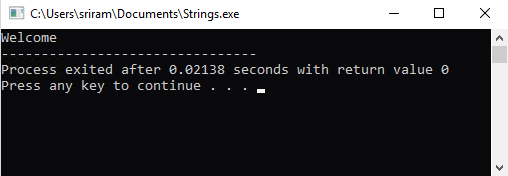## String Class

C++ does not support any built-in string types. In the previous example, we used the array of characters terminated by a \0 to store the string value and perform operations on it (e.g., print the value of the string). This type of string is called the C Strings. C++ offers a new string class very similar to the C Strings but with efficient programming capabilities. Internally, the working of the string class is the same as C Strings (by using a character array to store the characters), while the memory definition, allocation, and addition of null character at the end are all handled by the String class itself. In this section, we will take a detailed look into the different functions that are available as a part of the String class in C++.

While the character arrays as we have discussed above it is a statically allocated during the compile-time, while the strings are objects and hence can be dynamically allocated, Character arrays have few functions operating on them which can manipulate them. The string class has got set of various functions including the iterator functions that we will discuss in this article later on.

## String Functions

The different string functions in the String class are :

• Strcpy

• Strcat

• Strlen

• Strcmp

• Strchr

• Strstr

### Strcpy(s1, s2)

This string function is a standard function of C++ that is used to copy the string s2 into string s1. We need to pass two different string values to the function strcpy() to copy the string values as described in the below example.

Example Program
```
#include <iostream>
#include <cstring>
using namespace std;

int main()
{
char string1[]="Good";
char string2[]="Morning";
strcpy(string1, string2);
cout << "The result of strcpy is : " <<string1 << '\n';
}
```

The result of this program will be that the value of string2 (Morning) will be copied to string1 (Good) and string2 value will be printed (Morning).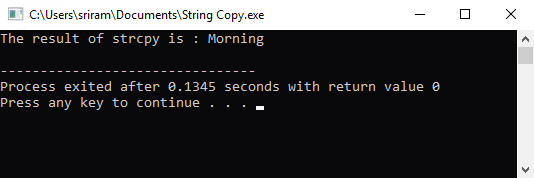### Strcat(s1, s2)

This string function is used to append the string value or concatenate the string s2 at the end of string s1. Below is a simple example where the two string values have been passed to the function strcat().

Example Program
```

#include <iostream>
#include <cstring>
using namespace std;

int main()
{
char string1[]="Good";
char string2[]="Morning";
strcat(string1, string2);
cout << "The result of strcat is : " <<string1 << '\n';
}
```

The result of this program will be that the value of string2 (Morning) will be concatenated to string1 (Good) and string2 value will be printed (GoodMorning).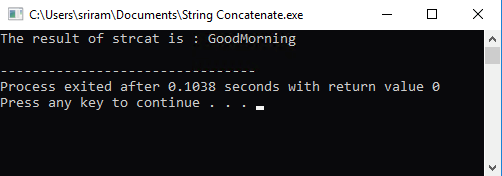### Strlen(s1)

This string function calculates and returns the length of the string s1.

Example Program
```
#include <iostream>
#include <cstring>
using namespace std;

int main()
{
char string1[]="Good Morning";
cout << "The result of strlen is : " <<strlen(string1)<< '\n';
}
```

The result of this program will be the length of string1 which will be 12.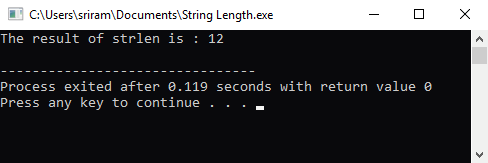### Strcmp(s1, s2)

This string function compares the two string values and returns 0 if the two strings are the same, -1 if the value of string1<string2, and 1 when string1>string2.

Example Program #1

```
#include <iostream>
#include <cstring>
using namespace std;

int main()
{
char string1[]="Good";
char string2[]="Morning";
cout << "The result of strcmp is : " <<strcmp(string1, string2) << '\n';
}
```

The result of this program for the following input will be -1.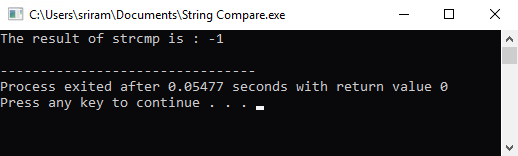Example Program #2
```

#include <iostream>
#include <cstring>
using namespace std;

int main()
{
char string1[]="Morning";
char string2[]="Good";
cout << "The result of strcmp is : " <<strcmp(string1, string2) << '\n';
}
```

The result of this program for the following input will be 1.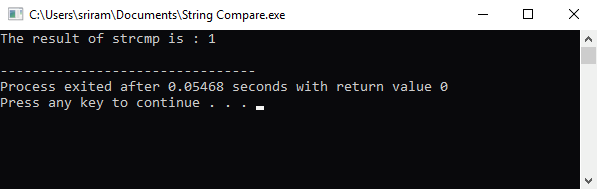Example Program #3
``` #include <iostream>
#include <cstring>
using namespace std;

int main()
{
char string1[]="Morning";
char string2[]="Morning";
cout << "The result of strcmp is : " <<strcmp(string1, string2) << '\n';
}
```

The result of this program for the following input will be 0.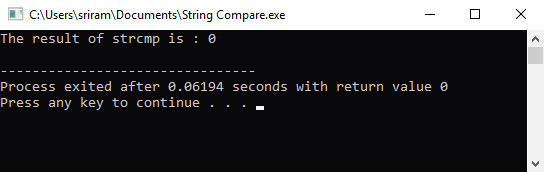### Strchr(s1, ch)

This string function searches for the character in the string and returns the pointer to the first occurrence of the character.

Example Program
```
#include <iostream>
#include <cstring>
using namespace std;

int main()
{
char string1[]="Good Morning";
char ch = 'a';
if (strchr(string1,ch))
cout << "The character "<< ch <<" is present in the string " << string1 << '\n';
else
cout << "The character "<< ch <<" is not present in the string " << string1 << '\n';
}
```

The output of this program will check to see if the character ‘a’ is present in the string ‘Good Morning’. The result will be printed as :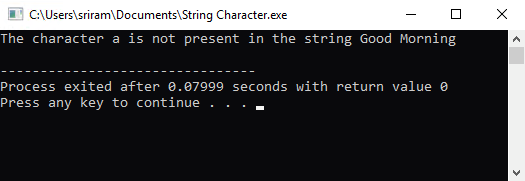If we change the character as ‘o’, it will return the pointer value of the first occurrence of the character o in the string Good Morning.

### Strstr(s1,s2)

This string function returns a pointer to the first occurrence of the string s2 in the string s1. Otherwise, the function returns a null pointer if s2 is not a part of s1.

Example Program
``` #include <iostream>
#include <cstring>
using namespace std;

int main()
{
char string1[]="Good Morning. How are you?";
char string2[] = "Good Morning";
char *ptr=strstr(string1,string2);
if (ptr)
cout << string2 <<" is present in the string " << string1 << '\n';
else
cout << string2 <<" is not present in the string " << string1 << '\n';
}
```

The result of this program will be :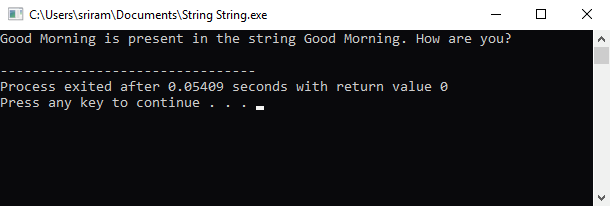##### Summary

In this detailed article, we have learned about the character array which is also called a C-style string along with the basics of the string class. String class comes with more utilities like iterator class as it’s a container class and also has discussed the variety of the string function to play with the string values.

In this section, we have covered the following concepts :

• Introduction to Strings

• What is a String Class?

• String Functions

• Types of String Functions with examples

Learn to Crack Your Technical Interview